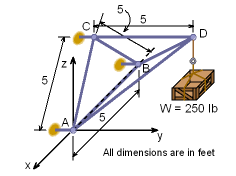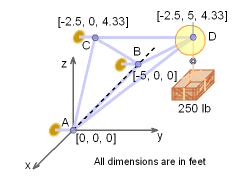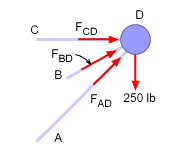Ch 6. Structures Multimedia Engineering Statics 2-D Trusses: Joints 2-D Trusses: Sections 3-D Trusses Frames and Machines
 Chapter 1. Basics 2. Vectors 3. Forces 4. Moments 5. Rigid Bodies 6. Structures 7. Centroids/Inertia 8. Internal Loads 9. Friction 10. Work & Energy Appendix Basic Math Units Sections Search eBooks Dynamics Fluids Math Mechanics Statics Thermodynamics Author(s): Kurt Gramoll ©Kurt GramollSTATICS- EXAMPLE Example3D Truss Lifting Crate Determine the force in the member CD, BD and AD of the space truss shown in the figure. Note that points A, B and C are in the x-z plane and member CD is parallel to y-axis. SolutionFirst, the co-ordinates of joints A, B, C and D need to be found. From the figure it can be seen that  ΔABC is an equilateral triangle with each side of 5 feet. The height of the equilateral triangle will be equal to 5 cos30o, or 4.330 feet. Thus, the coordinates each joint will be,      A = [0, 0, 0]               B = [-5, 0, 0]      C = [-2.5, 0, 4.33]     D = [-2.5, 5, 4.33] Since, there are only three unknown forces at joint D, the force analysis of the truss will begin at joint D. Recall, with 3D trusses, three equations can be used at each joint.Let the force in member AD, BD and CD be labeled as FAD, FBD and FCD respectively. Expressing each force acting at joint D in the vector notation gives,      W = -250 k      FAD = FAD (-2.5i + 5 j + 4.33k) / 7.071      FBD = FBD (2.5i + 5j + 4.33k) / 7.071      FCD = FCD 5j / 5 All forces have to be in equilibrium, giving      ΣF = 0      W + FAD + FBD + FCD = 0 Equating the i, j and k components gives,      ΣFx = 0     0.3536 FBD  - 0.3536 FAD = 0     FBD =  FAD      ΣFz = 0      0.6124 FAD + 0.6124 FBD - 250 = 0      By substituting FAD = FBD      0.6124 FAD + 0.6124 FAD - 250 = 0      FAD = 204.1 lb = FBD      ΣFy = 0      0.7071 FAD + 0.7071 FBD + FCD = 0      FCD = -288.6 lb Members AD and BD are in tension and member CD is in compression.

Practice Homework and Test problems now available in the 'Eng Statics' mobile app
Includes over 500 problems with complete detailed solutions.
Available now at the Google Play Store and Apple App Store.